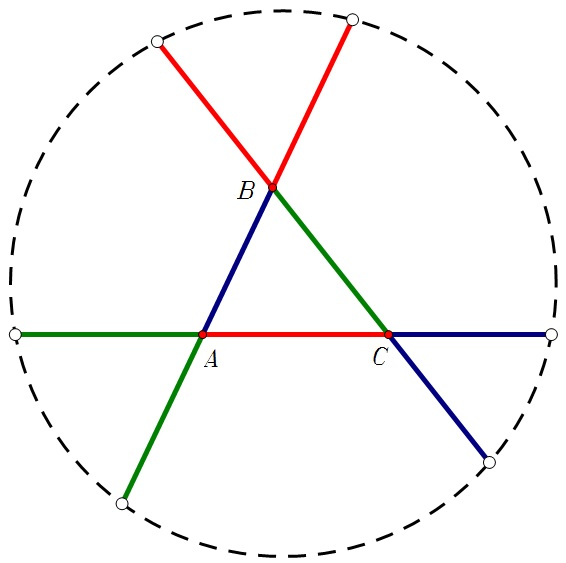# What Point Is This?

Geometry Level 3Let $ABC$ be any triangle, prolong the sides so that segments of the same color have the same length.

It can be shown that the six exterior points belong to the same circle $\Gamma$ [Try to prove this fact!]. Then complete the following sentence:

The center of $\Gamma$ is the $\ldots \ldots \ldots$ of triangle $ABC$.

×